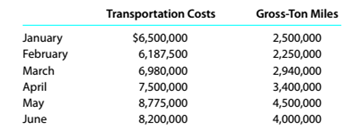# High-low method for service company Miss River Railroad decided to use the high-low method and operating data from the past six months to estimate the fixed and variable components of transportation costs. The activity base used by Miss River Railroad is a measure of railroad operating activity, termed "gross-ton miles," which is the total number of tons multiplied by the miles moved. Determine the variable cost per gross-ton mile and the fixed costs.### Survey of Accounting (Accounting I)

8th Edition
Carl Warren
Publisher: Cengage Learning
ISBN: 9781305961883### Survey of Accounting (Accounting I)

8th Edition
Carl Warren
Publisher: Cengage Learning
ISBN: 9781305961883

#### Solutions

Chapter
Section
Chapter 11, Problem 11.8E
Textbook Problem

## High-low method for service company Miss River Railroad decided to use the high-low method and operating data from the past six months to estimate the fixed and variable components of transportation costs. The activity base used by Miss River Railroad is a measure of railroad operating activity, termed "gross-ton miles," which is the total number of tons multiplied by the miles moved.Determine the variable cost per gross-ton mile and the fixed costs.

Expert Solution
To determine

Concept Introduction:

Mixed Costs:

There are three types of costs according to the unit of production; Variable, Fixed and Mixed. Variable costs change proportionally with the number of units produced and variable cost per unit remains constant. Fixed Cost remains same in totality irrespective of the number of units produced.

The mixed cost is the mix of variable and fixed cost, some of its part is fixed and some variable.

High-Low Method:

This method is used to determine the fixed as well as variable part of the mixed cost. The formula for calculation of variable cost per unit is as follows:

Variable cost per unit =  ( High Cost - Low Cost ) ( High units - low units )

The formula to calculate the Fixed Cost is as follows:

Fixed Cost = Total cost - (Units×Variable cost per unit)

To Calculate:

The variable cost per unit and total fixed cost

### Explanation of Solution

The variable cost per unit and total fixed cost are calculated as follows:

 High Low Method High Low Difference A B A-B Number of units 4500000

### Want to see the full answer?

Check out a sample textbook solution.See solution

### Want to see this answer and more?

Bartleby provides explanations to thousands of textbook problems written by our experts, many with advanced degrees!

See solution